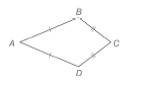Chapter 4.2, Problem 34EElementary Geometry For College St...

7th Edition
Alexander + 2 others
ISBN: 9781337614085

Solutions

Chapter
SectionElementary Geometry For College St...

7th Edition
Alexander + 2 others
ISBN: 9781337614085
Textbook Problem

For Exercises 32 to 35, consider kite A B C D with A B ¯ ≅ A D ¯ and B C ¯ ≅ D C ¯ .For kite A B C D , A B = B C + 5 . If the perimeter of A B C D is 59.2 , find B C .To determine

To find:

BC

Explanation

Calculation:

Given,

The kite ABCD with

BC¯DC¯,

AB=BC+5, and the perimeter of the kite =59.2

The perimeter of the kite ABCD is given by

P=2(AB+BC)

59.2=2BC+5+BC

59.2=2BC+BC+5

59

Still sussing out bartleby?

Check out a sample textbook solution.

See a sample solution

The Solution to Your Study Problems

Bartleby provides explanations to thousands of textbook problems written by our experts, many with advanced degrees!

Get Started

In Exercises 4756, solve the given equation for the indicated variable. 423x=256

Finite Mathematics and Applied Calculus (MindTap Course List)

Subtract and check: 60,0009,876_

Elementary Technical Mathematics

Sometimes, Always, or Never: fxy = fyx.

Study Guide for Stewart's Multivariable Calculus, 8th

Evaluate . e + 1

Study Guide for Stewart's Single Variable Calculus: Early Transcendentals, 8th